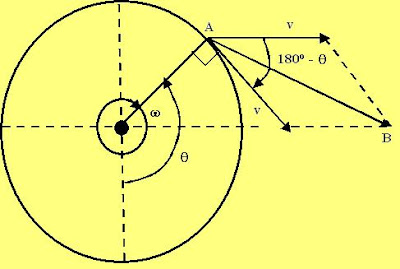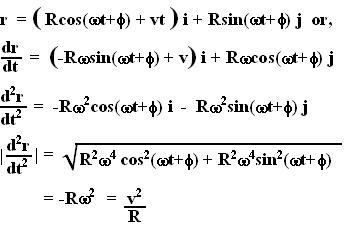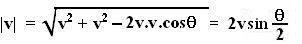## Friday, July 27, 2007

### Irodov Problem 1.52(a) This part does not need a lot of explanation I feel. We know that the wheel is moving at a constant velocity in the linear direction, thus there is no acceleration due to this motion. The point A however is moving in a circle, thus it will be undergoing the centripetal acceleration given by.
Nevertheless a completely mathematical treatment is possible and I shall provide it. It will also better explain the origin of centripetal acceleration. The position vector of a any point on the rim can be expressed as,(b) The distance traversed by the wheel rim is given by,. As seen in the figure, when the rim point is at an angle, the magnitude of the net velocity vector (AB in the figure), using basic trigonometry, is given by,Thus, the distance traveled by the rim of the wheel between two points of contact is given by,